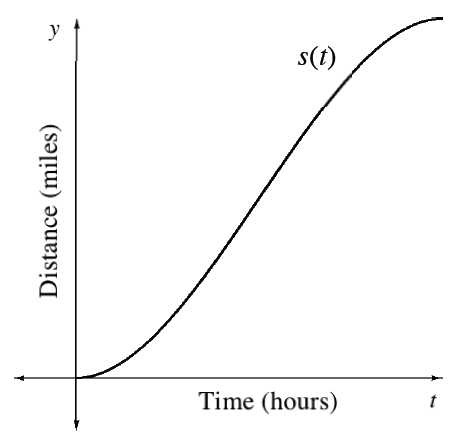### Home > APCALC > Chapter 4 > Lesson 4.2.2 > Problem4-59

4-59.

While driving to work, Camille decided to keep track of the time and the distance she traveled. Taking the data that she gathered, she found the function $s$ to represent her distance as a function of time:

If she drove $50$ miles in a total of $1$ hour, how fast did she travel on average?

$s\left(t\right) = –100t^{3} + 150t^{2}$

1. If Camille’s trip took $1$ hour, how far did she drive?

You are given the distance function, $s\left(t\right)$.

2. What was her average velocity?

In order to let Max Velocity $= v\left(t\right)$, you will need the equation for $v\left(t\right)$. How can you obtain a velocity equation from a position equation?

3. Use your graphing calculator to determine her maximum velocity. How far into the trip did she reach this speed?

Use the eTool below to examine the graph.
Click the link at right for the full version of the eTool: Calc 4-59 HW eTool